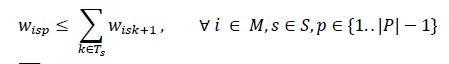# Formulating for loop for different indices on LHS and RHS

Hello

I have a constraint I am trying to formulate. But LHS and RHS use different indices.I have attached picture of mathematical formulation.

W is a binary variable indexed over i,s, and p which go from 1 to M (=2), 1 to S(=5), and 1 to P(=32). TA is an array which has different value for different s. For example, for k = TA{4}, k can take any value among 3,4, or 5. This is my current formulation:

``````for i = 1:M
for p = 1:P-1
for s = 1:S
for k = TA{s}
W(:,:,:) == sum (W(:,k,p+1));
end
end
end
end
``````

On LHS, i,s, and p start from 1 and go up to 2,5, and 31 respectively.
On RHS, i goes from 1 to 2, and p goes from 1 to 31. The variable is only summed over “k”, where k goes from TA{1} to TA{5}.

Since I have different indices on LHS and RHS, is it correct to define it this way? Or should I try to break this down into two equations?

Here is what it should evaluate to:

For i =1, s =1, p =1, k = TA{1} (where TA{1} = [1 2] which means k can be either 1 or 5)
W(1,1,1) == W(1,1,2) + W(1,5,2)

Which means if W is 1 for (1,1,1) then it will be 1 for either (1,1,2) or for (1,1,5).

Thank you for your time and I appreciate any feedback and advice on this!

Thank you.